# 8.5 统一机器人描述格式URDF

## 8.5.1 URDF基础

$\qquad$URDF（Unified Robot Description Format）统一机器人描述格式，URDF使用XML格式描述机器人文件。URDF语法规范，参考链接：http://wiki.ros.org/urdf/XML，URDF组件，是由不同的功能包和组件组成：$\qquad$其中urdf_parser和urder_interface已经在hydro之后的版本中去除了。urdf_paser_plugin是URDF基础的插件，衍生出了urdfdom（面向URDF文件）和collar_parser（面向相互文件）。在URDF当中，当你想要描述一个机器人的时候，例如小车的base_link和右轮right，两个link之间需要joint来连接。参考下图：

$\qquad \qquad \qquad \qquad \qquad \qquad \qquad \qquad$## 8.5.2 制作URDF模型

$\quad$（1）添加基本模型

$\quad$我们以构建一个小车为例子，为大家讲解这部分的内容:（相关的示例代码可以从我们的tf_demo中找到），我们的想法是，首先构建base_link作为小车的父坐标系，然后在base_link基础上，再构建左轮，右轮 和雷达 link. 最后不同的link之间通过joint来连接。参考代码如下：

$\quad \quad \quad \quad\quad \quad \quad \quad \quad \quad \quad$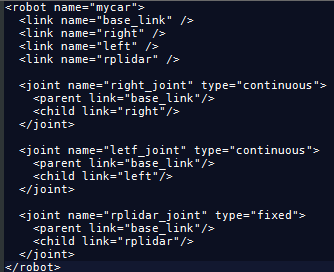$\quad$小技巧： sudo apt-get install liburdfdom-tools，安装完毕后，执行检查check_urdf my_car.urdf如果一切正常，就会有如下显示：

$\quad \quad \quad \quad\quad \quad \quad \quad \quad \quad \quad$$\quad \quad$随后打开新的终端，输入roslaunch urdf_demo display_urdf_link_joint.launch,回车之后,发现所有的link和joint都在一起了，详见urdf本章的demo。

$\quad \quad \quad \quad\quad \quad \quad \quad \quad \quad \quad\quad \quad \quad \quad \quad$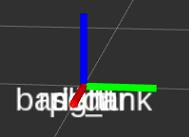$\quad$（2）添加机器人link之间的相对位置关系

$\quad$在基础模型之上，我们需要为机器人之间link来设相对位置和朝向的关系，URDF中通过<origin>来描述这种关系。

$\quad \quad \quad \quad\quad \quad \quad \quad \quad \quad \quad$$\quad \quad$随后打开新的终端，输入roslaunch urdf_demo display_urdf_link_position.launch,回车之后,发现所有的link和joint已经有在固定的位置上了，详见urdf本章的demo。

$\quad \quad \quad \quad\quad \quad \quad \quad \quad \quad \quad$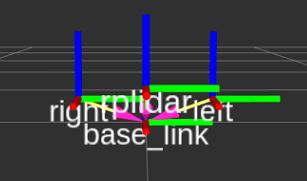$\quad$（3）添加模型的尺寸，形状和颜色等

$\quad$在已经设置好模型的link基础上，添加模型的形状（例如圆柱或长方体），相对于link的位置，颜色等。其中形状用<geometry>来描述，颜色用<color>来描述。

$\quad \quad \quad \quad\quad \quad \quad \quad \quad$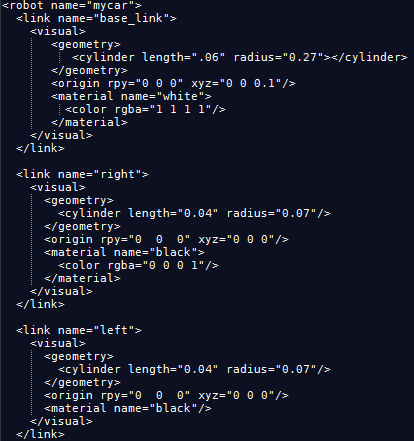$\quad$（4）显示URDF模型

$\quad$想要在rviz中显示出我们制作好的小车的URDF模型，可以写一个launch文件，参考如下：

$\quad \quad \quad \quad$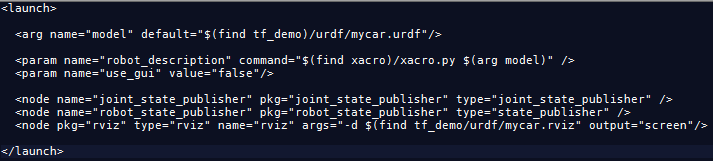$\quad$除了launch文件中的前3句，导入我们制作小车的URDF模型外，还需要添加joint_state_publisher和robot_state_publisjer这两个节点。效果如下：

$\quad \quad \quad \quad\quad \quad \quad \quad \quad$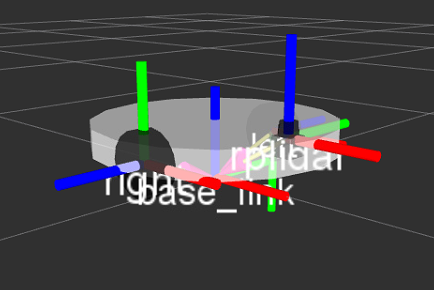$\quad$另外，我们可以输入rosrun rqt_tf_tree rqt_tf_tree,可以看到以下tf 树：$\quad$小技巧：你可以将launch文件中的param name="use_gui"的值由false改成true会弹出一个窗口，同一移动进度条，可以临时改变joint的朝向。

$\quad \quad \quad \quad\quad \quad \quad \quad \quad \quad \quad \quad \quad \quad\quad \quad \quad \quad \quad \quad \quad$## 8.5.3 制作xacro模型

$\quad$什么是Xacro? 我们可以把它理解成为针对URDF的扩展性和配置性而设计的宏语言(macro language)。有了Xacro，我们就可以像编程一样来写URDF文件。XACRO格式提供了一些更高级的方式来组织编辑机器人描述. 主要提供了三种方式来使得整个描述文件变得简单。

$\quad$（1）Constants

Usage：<xacro:property name="WIDTH" value="2.0"/>


$\quad$类似于C语言中的宏定义, 在头部定义后就可以${body_width}进行引用其数值，有了这个，至少我们可以把需要配置的变量进行统一管理和使用。 $\quad$（2）Macros Usage：<xacro:macro name="default_origin"> <origin xyz="0 0 0" rpy="0 0 0"/> </xacro:macro> <xacro:default_origin />  $\quad$Macros是xacro文件中最重要的部分. 就像宏函数一样, 完成一些最小模块的定义, 方便重用, 以及可以使用参数来标识不同的部分. $\quad$（3）Include $\quad$很多模型都是已宏的形式进行定义, 并以最小集团分成很多个文件. 而最终的机器人描述就变得非常简单了. 下面摘录一个ur5的描述文件. 从中可以看出来xacro的强大优势. 在最后的示例中我们还能够看到, urdf文件也是能够直接导入进来的. Usage：<?xml version="1.0"?> <robot xmlns:xacro="http://www.ros.org/wiki/xacro" name="ur5" > <!-- common stuff --> <xacro:include filename="$(find ur_description)/urdf/ur5/common.gazebo.xacro" />

<!-- ur5 -->
<xacro:include filename="$(find ur_description)/urdf/ur5/ur5.urdf.xacro" /> <!-- arm --> <xacro:ur5_robot prefix="" joint_limited="false"/> <link name="world" /> <joint name="world_joint" type="fixed"> <parent link="world" /> <child link = "base_link" /> <origin xyz="0.0 0.0 0.0" rpy="0.0 0.0 0.0" /> </joint> </robot>  $\quad$include类似于C语言中的include, 先将该文件扩展到包含的位置. 但包含进来的文件很有可能只是一个参数宏的定义. 并没有被调用.$\quad$ $\quad$举例说明打开新的终端，输入roslaunch urdf_demo display_xacro.launch,回车之后,发现所有的link和joint已经有在固定的位置上了，并且小车颜色和形状已经固定完成，详见urdf本章的demo。 $\quad \quad \quad \quad \quad \quad \quad \quad \quad \quad$## 8.5.4 制作gazebo模型 $\quad$在已经制作好的xcaro模型的基础上，添加gazebo模型的组建，看起已经变得十分的具有可操作性。对于二轮差动模型通过添加libgazebo_ros_diff_drive.so插件对小车左右轮的控制。 <gazebo> <plugin name="differential_drive_controller" filename="libgazebo_ros_diff_drive.so"> <robotNamespace>/</robotNamespace> <alwaysOn>true</alwaysOn> <legacyMode>false</legacyMode> <updateRate>50</updateRate> <leftJoint>mybot_left_wheel_hinge</leftJoint> <rightJoint>mybot_right_wheel_hinge</rightJoint> <wheelSeparation>${chassisWidth+wheelWidth}</wheelSeparation>
<torque>20</torque>
<commandTopic>mybot_cmd_vel</commandTopic>
<odometryTopic>mybot_odom</odometryTopic>
<odometryFrame>odom</odometryFrame>
</plugin>
</gazebo>


$\quad$通过添加libgazebo_ros_p3d.so来计算里程。

<gazebo>
<plugin name="ground_truth" filename="libgazebo_ros_p3d.so">
<frameName>map</frameName>
<bodyName>mybot_chassis</bodyName>
<topicName>odom</topicName>
<updateRate>30.0</updateRate>
</plugin>
</gazebo>


$\quad$最后，对gazebo模型中小车左右轮相关PID等参数进行设置

<gazebo reference="mybot_chassis">
<material>Gazebo/Orange</material>
</gazebo>
<gazebo reference="caster_wheel">
<mu1>0.0</mu1>
<mu2>0.0</mu2>
<material>Gazebo/Red</material>
</gazebo>
<gazebo reference="right_wheel">
<mu1 value="1.0"/>
<mu2 value="1.0"/>
<kp  value="10000000.0" />
<kd  value="1.0" />
<fdir1 value="1 0 0"/>
<material>Gazebo/Black</material>
</gazebo>
<gazebo reference="left_wheel">
<mu1 value="1.0"/>
<mu2 value="1.0"/>
<kp  value="10000000.0" />
<kd  value="1.0" />
<fdir1 value="1 0 0"/>
<material>Gazebo/Black</material>
</gazebo>

</robot>


$\quad$举例说明打开新的终端，输入roslaunch urdf_demo display_gazebo_rviz.launch,回车之后,发现所有的link和joint已经有在固定的位置上了，并且小车颜色和形状已经固定完成,gazebo界面同样显示正常，详见urdf本章的demo。